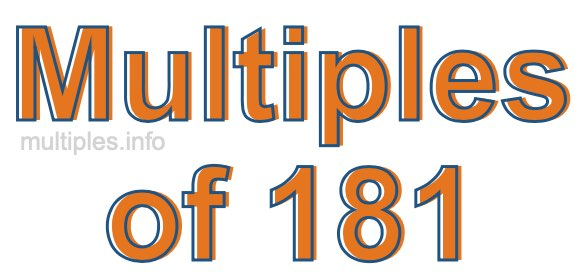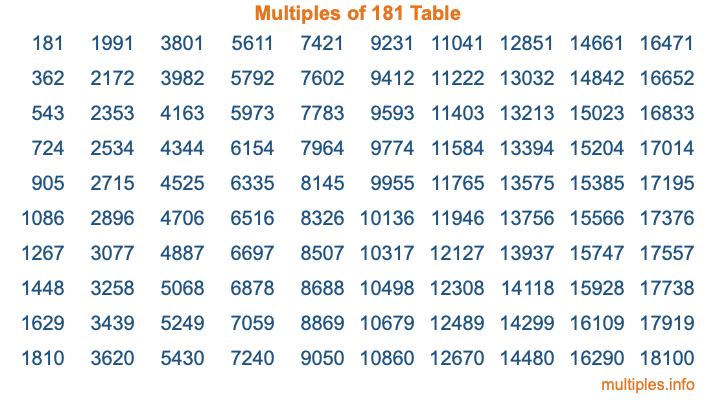Multiples of 181Welcome to the Multiples of 181 page. Here we will first teach you everything you will ever need to know about the multiples of 181, and then give you a study guide summary of everything we taught you to make sure you remember it all. Use this page to look up facts and learn information about the multiples of 181. This page will make you a multiples of one hundred eighty-one expert!

Definition of Multiples of 181
Multiples of 181 are all the numbers that when divided by 181 equal an integer. Each of the multiples of 181 are called a multiple. A multiple of 181 is created by multiplying 181 by an integer.

Therefore, to create a list of multiples of 181, you start with 1 multiplied by 181, then 2 multiplied by 181, then 3 multiplied by 181, and so on for as long as you want. Thus, the list of the first five multiples of 181 is 181, 362, 543, 724, and 905. To see a larger list of multiples of 181, see the printable image of Multiples of 181 further down on this page. We also have a category where you can choose any nth multiple of 181.

Multiples of 181 Checker
The Multiples of 181 Checker below checks to see if any number of your choice is a multiple of 181. In other words, it checks to see if there is any number (integer) that when multiplied by 181 will equal your number. To do that, we divide your number by 181. If the the quotient is an integer, then your number is a multiple of 181.

Is  a multiple of 181?

Least Common Multiple of 181 and ...
A Least Common Multiple (LCM) is the lowest multiple that two or more numbers have in common. This is also called the smallest common multiple or lowest common multiple and is useful to know when you are adding our subtracting fractions. Enter one or more numbers below (181 is already entered) to find the LCM.

Check out our LCM Calculator if you need more details about the Least Common Multiple or if you need the LCM for different numbers for adding and subtraction fractions.

nth Multiple of 181
As we stated above, 181 is the first multiple of 181, 362 is the second multiple of 181, 543 is the third multiple of 181, and so on. Enter a number below to find the nth multiple of 181.

th multiple of 181

Multiples of 181 vs Factors of 181
181 is a multiple of 181 and a factor of 181, but that is where the similarities end. All postive multiples of 181 are 181 or greater than 181. All positive factors of 181 are 181 or less than 181.

Below is the beginning list of multiples of 181 and the factors of 181 so you can compare:

Multiples of 181: 181, 362, 543, 724, 905, etc.

Factors of 181: 1, 181

As you can see, the multiples of 181 are all the numbers that you can divide by 181 to get a whole number. The factors of 181, on the other hand, are all the whole numbers that you can multiply by another whole number to get 181.

It's also interesting to note that if a number (x) is a factor of 181, then 181 will also be a multiple of that number (x).

Multiples of 181 vs Divisors of 181
The divisors of 181 are all the integers that 181 can be divided by evenly. Below is a list of the divisors of 181.

Divisors of 181: 1, 181

The interesting thing to note here is that if you take any multiple of 181 and divide it by a divisor of 181, you will see that the quotient is an integer.

Multiples of 181 Table
Below is an image of the first 100 multiples of 181 in a table. The table is in chronological order, column by column. The first column has the first ten multiples of 181, the second column has the next ten multiples of 181, and so on.The Multiples of 181 Table is also referred to as the 181 Times Table or Times Table of 181. You are welcome to print out our table for your studies.

Negative Multiples of 181
Although not often discussed or needed in math, it is worth mentioning that you can make a list of negative multiples of 181 by multiplying 181 by -1, then by -2, then by -3, and so on, to get the following list of negative multiples of 181:

-181, -362, -543, -724, -905, etc.

Multiples of 181 Summary
Below is a summary of important Multiples of 181 facts that we have discussed on this page. To retain the knowledge on this page, we recommend that you read through the summary and explain to yourself or a study partner why they hold true.

There are an infinite number of multiples of 181.

A multiple of 181 divided by 181 will equal a whole number.

181 divided by a factor of 181 equals a divisor of 181.

The nth multiple of 181 is n times 181.

The largest factor of 181 is equal to the first positive multiple of 181.

181 is a multiple of every factor of 181.

181 is a multiple of 181.

A multiple of 181 divided by a divisor of 181 equals an integer.

181 divided by a divisor of 181 equals a factor of 181.

Any integer times 181 will equal a multiple of 181.

Multiples of a Number
Here you can get the multiples of another number, all with the same attention to detail as we did for multiples of 181 on this page.

Multiples of
Multiples of 182
Did you find our page about multiples of one hundred eighty-one educational? Do you want more knowledge? Check out the multiples of the next number on our list!